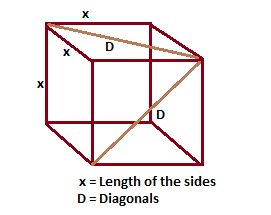# Diagonal of a Cube Formula

A cube is a three-dimensional solid figure. A cube is also known as the square solid that has edges with all the same length. In other words, the length, width, and height are equal, and each of its faces is a square. The main diagonal of a cube is the one that cuts through the centre of the cube; the diagonal of a face of a cube is not the main diagonal. The main diagonal of any cube can be found by multiplying the length of one side by the square root of 3.$\LARGE Diagonal\;of\;a\;Cube=\sqrt{3}x$
Where “x” is the cube side.

### Solved Examples

Question 1: Find the diagonal of the cube with the given side 5 cm?

Solution:

Given: side x = 5 cm

Use diagonal formula,

Diagonal of cube =

$$\begin{array}{l}\sqrt{3}x\end{array}$$

Diagonal of cube =

$$\begin{array}{l}\sqrt{3}*5\end{array}$$

We know that the value of √3 is 1.734

Therefore, the diagonal of a cube  = 1.734 (5)

Diagonal of cube = 8.67 cm